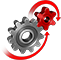# WolframSystemModeler

## Boost Converter

Boost converters are used to step up voltage from a lower level to a higher level. Boost converters can be found in electrical vehicles to increase the voltage from a battery stack to the engine and in LED lights to increase the voltage from a battery cell to the light diode.

To run this example, you'll need

### The Model

A boost converter is a switched-mode power supply that uses two switches (usually a diode and a transistor), an inductor, and a capacitor to convert direct current voltage from a lower to a higher level. When the switch is turned off, the inductor current goes through the diode, the load, and the capacitor. When the switch is turned on, the inductor current increases and doesn’t pass through the diode. A filter capacitor is added to smooth out the output voltage.A model of a boost converter created in System Modeler from standard components.

Show parameter values with units

Quickly check the values of your parameters, which are rendered using your chosen display unit.

### Current Ripple Analysis

A boost converter has a pulsating current on the output. Output ripple is one of the main disadvantages of using a switched-mode power supply.The generated output voltage, and the target voltage.The inductor current is the same as the input current.
The output voltage is always higher than the input, and the output current is always lower than the input current.The min and max values marked in the inductor current.

Mix analytical and simulation analysis

The theoretical value for the ripple in the current,, can be compared to the simulated values.

Using the Wolfram Language, the max and min can be retrieved from the simulation data, and the peak-to-peak current ripple can be calculated. In this case it is 0.0019. This value can be compared to the theoretical value of 0.002. The downloadable example contains this calculation.

Model and simulate efficient switched‐mode power converters

## Wolfram System Modeler

Questions? Comments? Contact a Wolfram expert »